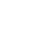# Dijkstra’s Algorithm in KotlinAlex Woods

April 27, 2019

What is the shortest path from a start vertex to every other vertex in the graph?

This is useful—we need to do things like route messages through a phone network, and find the best route from point A to point B.

It hinges on the observation that any subpath of a shortest path must also be a shortest path (I encourage you to think about why). Here is my favorite explanation of it, and here is a useful visualization.

My code is more focused on education, mirroring the way I learned it originally at GT. To speed it up, you can make the operation of getting the next vertex to explore (`v`, in the code) done with a min heap.

Some other good implementations to compare with are Rosetta code, and the one in Kotlin Algorithm Club (what an awesome project!).

So, first let’s write a Graph class, so the algorithm has something to act upon.

``````data class Graph<T>(
val vertices: Set<T>,
val edges: Map<T, Set<T>>,
val weights: Map<Pair<T, T>, Int>
)
``````

Without going too much into the details of initialization and providing a simple API, this is essentially my Graph class (on Github I add an additional constructor).

Now, let’s actually take a look at the algorithm.

``````fun <T> dijkstra(graph: Graph<T>, start: T): Map<T, T?> {
val S: MutableSet<T> = mutableSetOf() // a subset of vertices, for which we know the true distance

val delta = graph.vertices.map { it to Int.MAX_VALUE }.toMap().toMutableMap()
delta[start] = 0

val previous: MutableMap<T, T?> = graph.vertices.map { it to null }.toMap().toMutableMap()

while (S != graph.vertices) {
val v: T = delta
.filter { !S.contains(it.key) }
.minBy { it.value }!!
.key

graph.edges.getValue(v).minus(S).forEach { neighbor ->
val newPath = delta.getValue(v) + graph.weights.getValue(Pair(v, neighbor))

if (newPath < delta.getValue(neighbor)) {
delta[neighbor] = newPath
previous[neighbor] = v
}
}

}

return previous.toMap()
}

fun <T> shortestPath(shortestPathTree: Map<T, T?>, start: T, end: T): List<T> {
fun pathTo(start: T, end: T): List<T> {
if (shortestPathTree[end] == null) return listOf(end)
return listOf(pathTo(start, shortestPathTree[end]!!), listOf(end)).flatten()
}

return pathTo(start, end)
}
``````

Man, Kotlin has fantastic API's for functional programming.

Here's the test:

``````@Test
fun shouldCalculateCorrectShortestPaths() {
val weights = mapOf(
Pair("A", "B") to 2,
Pair("A", "C") to 8,
Pair("A", "D") to 5,
Pair("B", "C") to 1,
Pair("C", "E") to 3,
Pair("D", "E") to 2
)

val start = "A"
val shortestPathTree = dijkstra(Graph(weights), start)

Assert.assertEquals(listOf(start, "B", "C"), shortestPath(shortestPathTree, start, "C"))
Assert.assertEquals(listOf(start, "B", "C", "E"), shortestPath(shortestPathTree, start, "E"))
Assert.assertEquals(listOf(start, "D"), shortestPath(shortestPathTree, start, "D"))
}
``````

If you’d like to run the code, clone the git repo and get started using gradle.

Want to know when I write a new article?

Get new posts in your inbox